# I trained a Network to Speak Like Me

8 minute read

Over the course of the past months, I wrote over 100 articles on my blog. That’s quite a large amount of content. An idea then came to my mind : train a language generation model to speak like me. Or more specifically, to write like me. This is the perfect way to illustrate the main concepts of language generation, its implementation using Keras, and the limits of my model.

I have found this Kaggle Kernel to be a useful resource.

# Language generation

Language Generation is a subfield of Natural Language Processing that aims to generate meaningful textual content. Most often, the content is generated as a sequence of individual words.

For the big idea, here is how it works :

• you train a model to predict the next word of a sequence
• you give the trained model an input
• and iterate N times so that it generates the next N words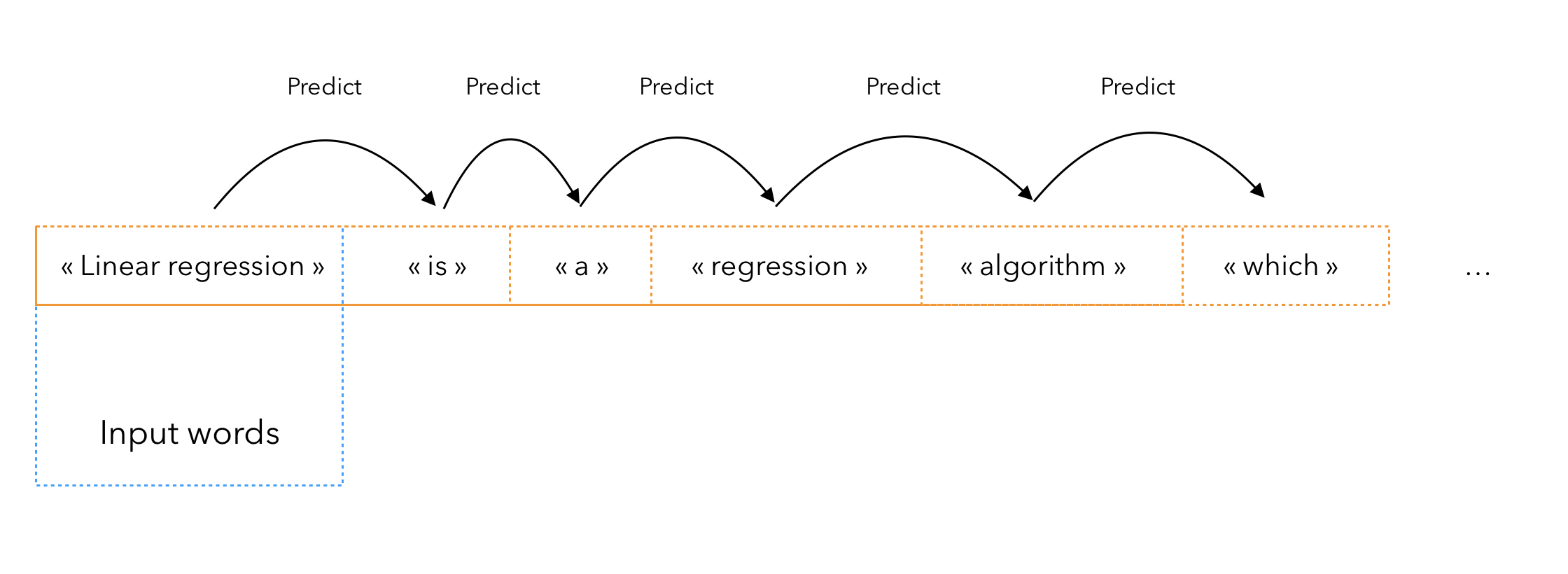## Dataset Creation

The first step is to build a dataset that can be understood by the network we are later on going to build. Start by importing the following packages :

``````from keras.preprocessing.sequence import pad_sequences
from keras.layers import Embedding, LSTM, Dense, Dropout
from keras.preprocessing.text import Tokenizer
from keras.callbacks import EarlyStopping
from keras.models import Sequential
import keras.utils as ku

import pandas as pd
import numpy as np
import string, os
``````

### Load the data

The header of each and every article I have written follows this template :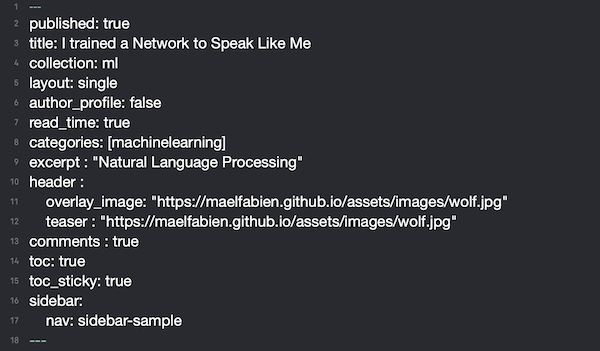This is the type of content we would typically not like to have in our final dataset. We will instead focus on the text itself. First, we need to point to the folder that contains the articles :

``````import glob, os

os.chdir("/MYFOLDER/maelfabien.github.io/_posts/")
``````

### Sentence Tokenizing

Then, open each article, and append the content of each article to a list. However, since our aim is to generate sentences, and not whole articles (so far…), we will split each article into a list of sentences, and append each sentences to the list `all_sentences` :

``````all_sentences= []

for file in glob.glob("*.md"):
f = open(file,'r')
txt = f.read().replace("\n", " ")
try:
sent_text = nltk.sent_tokenize(''.join(txt.split("---")).strip())
for k in sent_text :
all_sentences.append(k)
except :
pass
``````

Overall, we have a little more than 6’800 training sentences :

``````len(all_sentences)
``````

`6858`

The process so far is the following :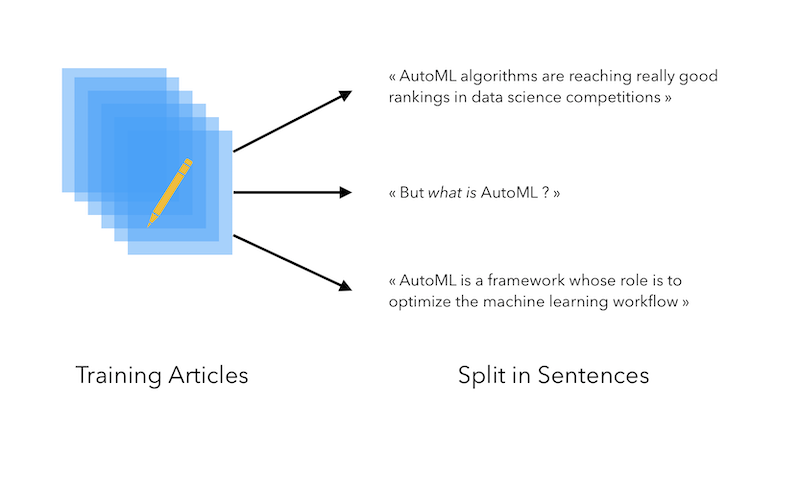### N-gram creation

Then, the idea is to create N-grams of words that occur together. To do so, we need to :

• fit a tokenizer on the corpus to associate an index to each token
• break down each sentence in the corpus as a sequence of tokens
• store sequences of tokens that happens together

It can be illustrated in the following way :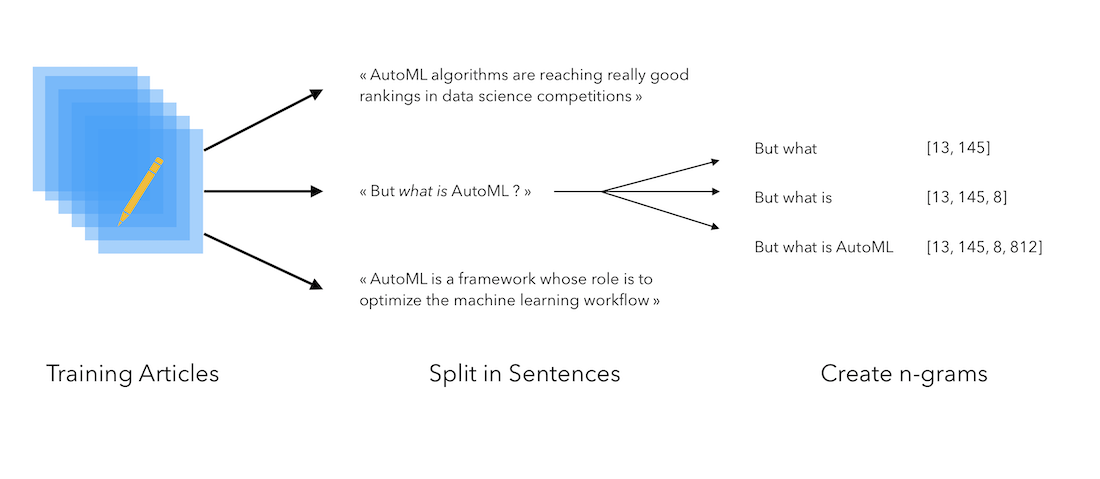Let’s implement this. We first need to fit the tokenizer :

``````tokenizer = Tokenizer()
tokenizer.fit_on_texts(all_sentences)
total_words = len(tokenizer.word_index) + 1
``````

The variable `total_words` contains the total number of different words that have been used. Here, 8976. Then, for each sentence, get the corresponding tokens and generate the N-grams :

``````input_sequences = []
for sent in all_sentences:
token_list = tokenizer.texts_to_sequences([sent])
for i in range(1, len(token_list)):
n_gram_sequence = token_list[:i+1]
input_sequences.append(n_gram_sequence)
``````

The `token_list` variable contains the sentence as a sequence of tokens :

``````[656, 6, 3, 2284, 6, 3, 86, 1283, 640, 1193, 319]
[33, 6, 3345, 1007, 7, 388, 5, 2128, 1194, 62, 2731]
[7, 17, 152, 97, 1165, 1, 762, 1095, 1343, 4, 656]
``````

Then, the `n_gram_sequences` creates the n-grams. It starts with the first two words, and then gradually adds words :

``````[656, 6]
[656, 6, 3]
[656, 6, 3, 2284]
[656, 6, 3, 2284, 6]
[656, 6, 3, 2284, 6, 3]
...
``````

### Padding

We are now facing the following problem : not all sequences have the same length ! How can we solve this ?

We will use paddding. Paddings adds sequences of 0’s before each line of the variable `input_sequences` so that each line has the same length as the longest line.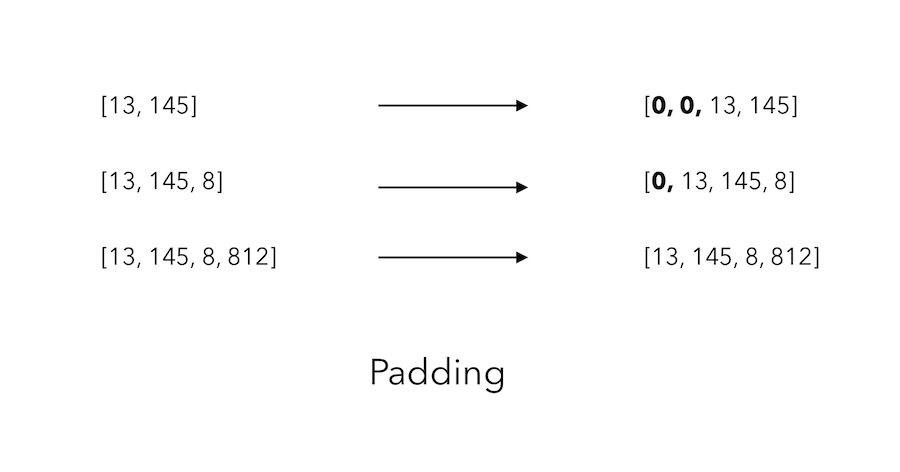In order to pad all sentences to the maximum length of the sentences, we must first find the longest sentence :

``````max_sequence_len = max([len(x) for x in input_sequences])
``````

It is equal to `792` in my case. Well, that looks quite large for a single sentence ! Since my blog contains some code and tutorials, I expect this single sentence to actually by Python code. Let’s plot the histogram of the length of the sequences :

``````import matplotlib.pyplot as plt

plt.figure(figsize=(12,8))
plt.hist([len(x) for x in input_sequences], bins=50)
plt.axvline(max_sequence_len, c="r")
plt.title("Sequence Length")
plt.show()
``````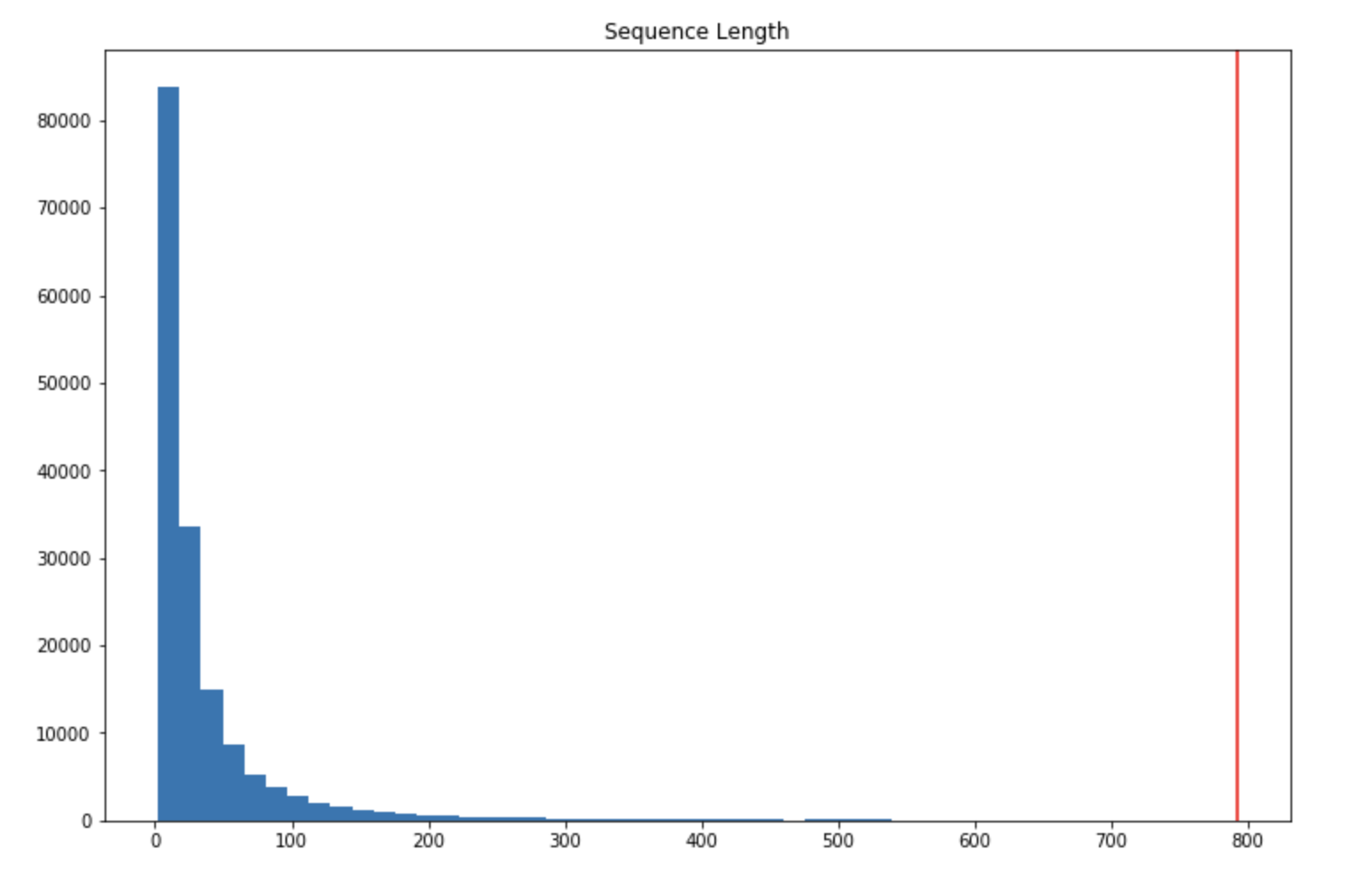There are indeed very few examples with 200 + words in a single sequence. How about setting the maximal sequence length to 200 ?

``````max_sequence_len = 200
input_sequences = np.array(pad_sequences(input_sequences, maxlen=max_sequence_len, padding='pre'))
``````

It returns something like :

``````array([[   0,    0,    0, ...,    0,  656,    6],
[   0,    0,    0, ...,  656,    6,    3],
[   0,    0,    0, ...,    6,    3, 2284],
...,
``````

### Split X and y

We now have fixed length arrays, most of them are filled with 0’s before the actual sequence. Right, how do we turn that into a training set? We need to split X and y! Remember that our aim is to predict the next word of a sequence. We must therefore takes all tokens except for the last one as our `X`, and take the last one as our `y`.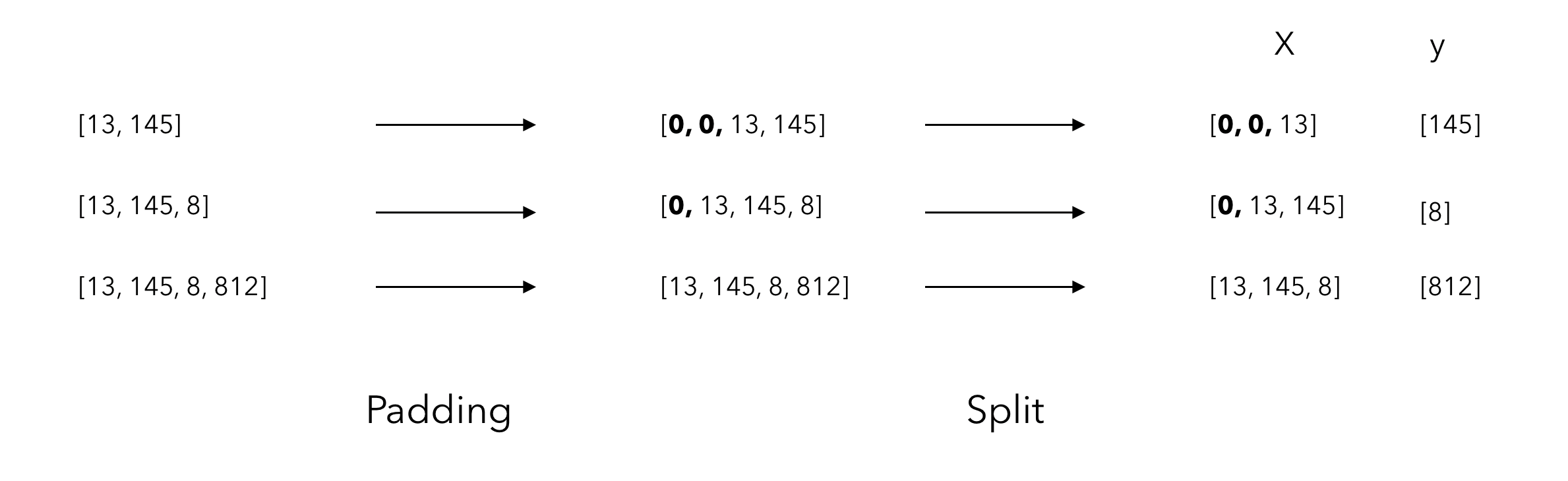In Python, it’s as simple as that :

``````X, y = input_sequences[:,:-1],input_sequences[:,-1]
``````

We will now see this problem as a multi-class classification task. As usual, we must first one-hot encode the `y` to get a sparse matrix that contains a 1 in the column that corresponds to the token, and 0 eslewhere :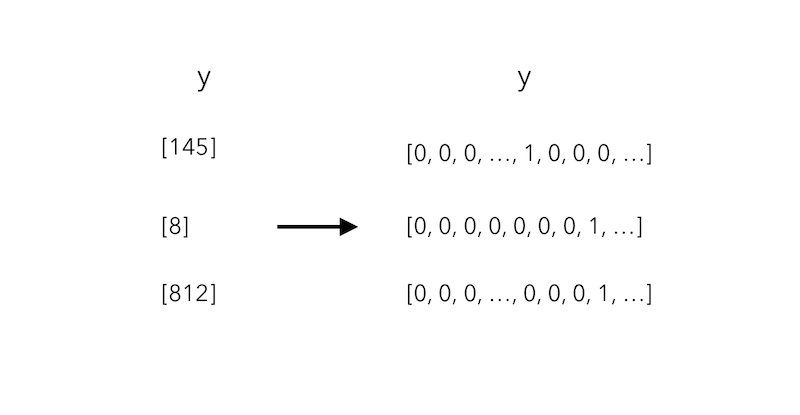In Python, using keras utils `to_categorical` :

``````y = ku.to_categorical(y, num_classes=total_words)
``````

Lets us now check the sizes of `X` and `y` :

``````X.shape
``````

`(164496, 199)`

``````y.shape
``````

`(164496, 8976)`

We have 165’000 training samples. X is 199 columns wide since it corresponds to the longest sequence we allow (200) minus one, the label to predict. Y has 8976 columns, which corresponds to a sparse matrix of all the vocabulary words. The dataset is now ready !

## Build the model

We will be using Long Short-Term Memory networks (LSTM). LSTM have the important advantage of being able to understand depenence over a whole sequence, and therefore, the beginning of a sentence might have an impact on the 15th word to predict. On the other hand, Recurrent Neural Networks (RNN) only imply a dependence on the previous state of the network, and only the previous word would help predict the next one. We would quickly miss context if we chose RNNs, and therefore, LSTMs seem to be the right choice.

### Model architecture

Since the training can be very (very) (very) (very) (very) (no joke) long, we will build a simple 1 Embedding + 1 LSTM layer + 1 Dense network :

``````def create_model(max_sequence_len, total_words):

input_len = max_sequence_len - 1

model = Sequential()

# Add Input Embedding Layer
model.add(Embedding(total_words, 10, input_length=input_len))

# Add Hidden Layer 1 - LSTM Layer
model.add(LSTM(100))
model.add(Dropout(0.1))

# Add Output Layer
model.add(Dense(total_words, activation='softmax'))

model.compile(loss='categorical_crossentropy', optimizer='adam')

return model

model = create_model(max_sequence_len, total_words)
model.summary()
``````

First, we add an embedding layer. We pass that into an LSTM with 100 neurons, add a dropout to control neuron co-adaptation, and end with a dense layer. Notice that we apply a softmax activation function on the last layer to get the probability that the output belongs to each class. The loss used is the categorical cross-entropy, since it is a multi-class classification problem.

The summary of the model is :

``````_________________________________________________________________
Layer (type)                 Output Shape              Param #
=================================================================
embedding_2 (Embedding)      (None, 199, 10)           89760
_________________________________________________________________
lstm_2 (LSTM)                (None, 100)               44400
_________________________________________________________________
dropout_2 (Dropout)          (None, 100)               0
_________________________________________________________________
dense_2 (Dense)              (None, 8976)              906576
=================================================================
Total params: 1,040,736
Trainable params: 1,040,736
Non-trainable params: 0
____________________________

``````

### Train the model

We are now (finally) ready to train the model !

``````model.fit(X, y, batch_size=256, epochs=100, verbose=True)
``````

The training of the model will then start :

``````Epoch 1/10
164496/164496 [==============================] - 471s 3ms/step - loss: 7.0687
Epoch 2/10
73216/164496 [============>.................] - ETA: 5:12 - loss: 7.0513
``````

On a CPU, a single epoch takes around 8 minutes. On a GPU, you should modify the Keras LSTM network used since it cannot be used on GPU. You would instead need this :

``````# Modify Import
from keras.layers import Embedding, LSTM, Dense, Dropout, CuDNNLSTM

# In the Moddel
...
model.add(CuDNNLSTM(100))
...
``````

This reduces training time to 2 minutes per epoch, which makes it acceptable. I have personnaly trained this model on Google Colab. I tend to stop the training at several steps to make so sample predictions and control the quality of the model given several values of the cross entropy.

Here are my observations :

Loss Value Sentence Generated
± 7 Generates only the word “The” since most frequent
± 4 Easily falls into cyclical patterns if the same word occurs twice
± 2.8 Becomes interesting e.g “Machine” inputs leads to “Learning algorithms …”

## Generating sequences

If you have read the article up to here, you basically came here for that : generate sentences ! To generate sentences, we need to apply the same transformations to the input text. We will build a loop that generates for a given number of iterations the next word :

``````input_txt = "Machine"

for _ in range(10):

# Get tokens
token_list = tokenizer.texts_to_sequences([input_txt])
# Pad the sequence
token_list = pad_sequences([token_list], maxlen=max_sequence_len-1, padding='pre')
# Predict the class
predicted = model.predict_classes(token_list, verbose=0)

output_word = ""

# Get the corresponding work
for word,index in tokenizer.word_index.items():
if index == predicted:
output_word = word
break

input_txt += " "+output_word
``````

When the loss is around 3.1, here is the sentence it generates with “Google” as an input :

`Google is a large amount of data produced worldwide`

It does not really mean anything, but it sucessfully associates Google to the notion of large amount of data. It’s quite impressive since it simply relies on the co-occurence of words, and does not integrate any grammatical notion. If we wait a bit longer in the training and let the loss decrease to 2.6, and give it the input “In this article” :

`In this article we'll cover the main concepts of the data and the dwell time is proposed mentioning the number of nodes`

I hope this article was useful. I have tried to illustrate the main concepts, challenges and limits of language generation. Larger networks and transfer learning are definitely sources of improvement compared to the approach we discussed in this article. Please leave a comment if you have any question :)

Sources :

Categories:

Updated: